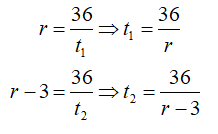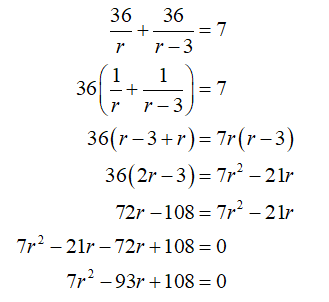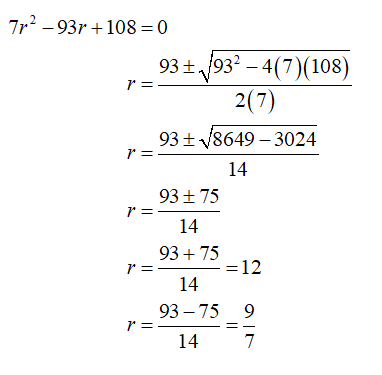# Naoki bikes the 36 mi to Hillsboro averaging a certain speed. The return trip is made at a speed that is 3 mph slower. Total time for the round trip is 7 hr. Find Naoki’s average speed on each part of the trip.Use rt = d

Question
1 views

Naoki bikes the 36 mi to Hillsboro averaging a certain speed. The return trip is made at a speed that is 3 mph slower. Total time for the round trip is 7 hr. Find Naoki’s average speed on each part of the trip.

Use rt = d

check_circle

Step 1

According to the given information, the distance Naoki travels to Hillsboro is 36 mi. Total time for the round trip is 7 hours. The return trip is made at a speed that is 3mph slower.

So, by using the formula rate(r) = distance (d) / time (t)Step 2

Now, the total time is 7 hours. So,Step 3

Now, solve the quadratic equation using discriminate method....

### Want to see the full answer?

See Solution

#### Want to see this answer and more?

Solutions are written by subject experts who are available 24/7. Questions are typically answered within 1 hour.*

See Solution
*Response times may vary by subject and question.
Tagged in